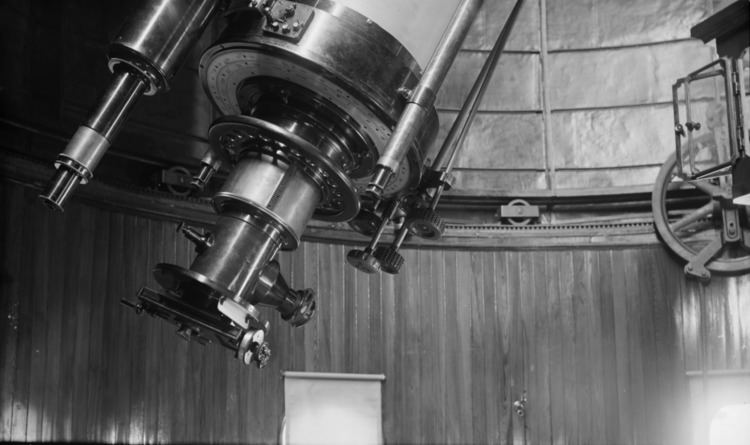# Filar micrometer

Updated onA filar micrometer is a specialized eyepiece used in astronomical telescopes for astrometry measurements, in microscopes for specimen measurements, and in alignment and surveying telescopes for measuring angles and distances on nearby objects. The word filar derives from Latin filum, meaning 'a thread'. It refers to the fine threads or wires used in the device.

## Construction and use

A typical filar micrometer consists of a micrometer and a reticle that has two fine parallel wires or threads that can be moved by the observer using a micrometer screw mechanism. The wires are placed in the focal image plane of the eyepiece so they remain sharply superimposed over the object under observation, while the micrometer motion moves the wires across the focal plane. Other designs employ a fixed reticle, against which one wire or a second reticle moves. By rotating the eyepiece assembly in the eyetube, the measurement axis can be aligned to match the orientation of the two points of observation.

At one time, it was common to use spider silk as a thread.

By placing one wire over one point of interest and moving the other to a second point, the distance between the two wires can be measured with the micrometer portion of the instrument. Given this precise distance measurement at the image plane, a trigonometric calculation with the objective focal length yields the angular distance between the two points seen in a telescope. In a microscope, a similar calculation yields the spatial distance between two points on a specimen.

In an alignment telescope, the precise micrometric measurement of the eyepiece image directly indicates the real distance of a nearby observed point from the line of sight. This absolute measurement is independent of the distance to the object, due to the telecentricity principle.

A common use of filar micrometers in astronomical telescopes was measuring the distance between double stars.

Filar micrometers are little used in modern astronomy, having been replaced by digital photographic techniques where digital pixels provide a precise reference for image distance. However, filar eyepieces are still used in teaching astronomy and by some amateur astronomers.

## Prior devices

The precursor to the filar micrometer was the micrometer eyepiece, invented by William Gascoigne.

Earlier measures of angular distances relied on inserting into the eyepiece a thin metal sheet cut in the shape of a narrow, isosceles triangle. The sheet was pushed into the eyepiece until the two adjacent edges of the metal sheet simultaneously occulted the two objects of interest. By carefully measuring the position where the objects were extinguished and knowing the focal length of the eyepiece, the angular distance could be calculated. Christiaan Huygens used such a device.

Topics